• 收起
• 向左旋转
• 向右旋转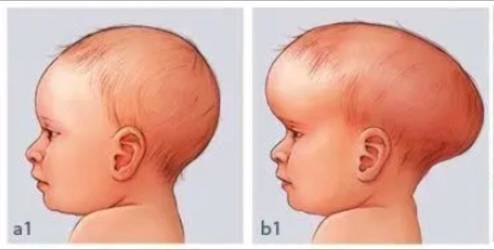• 收起
• 向左旋转
• 向右旋转• 收起
• 向左旋转
• 向右旋转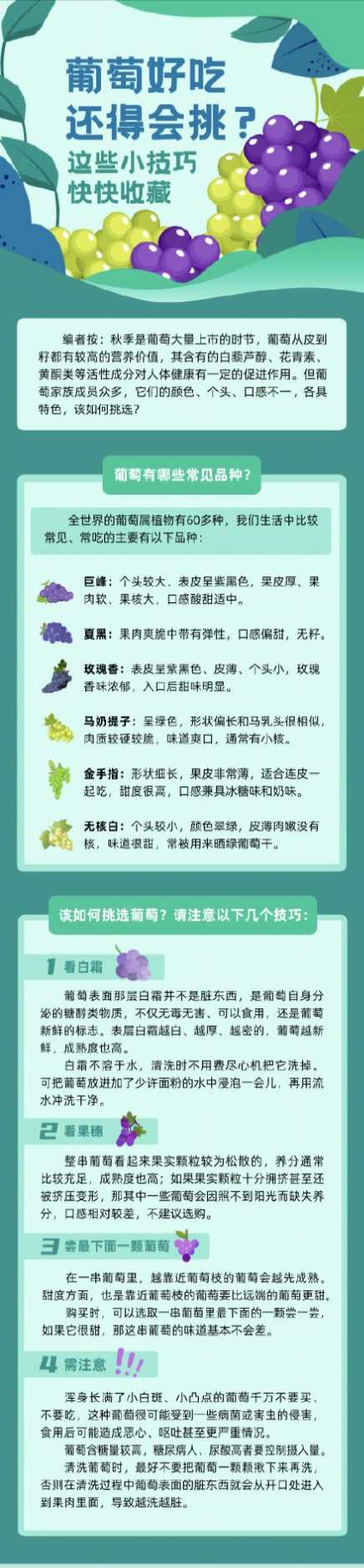• 收起
• 向左旋转
• 向右旋转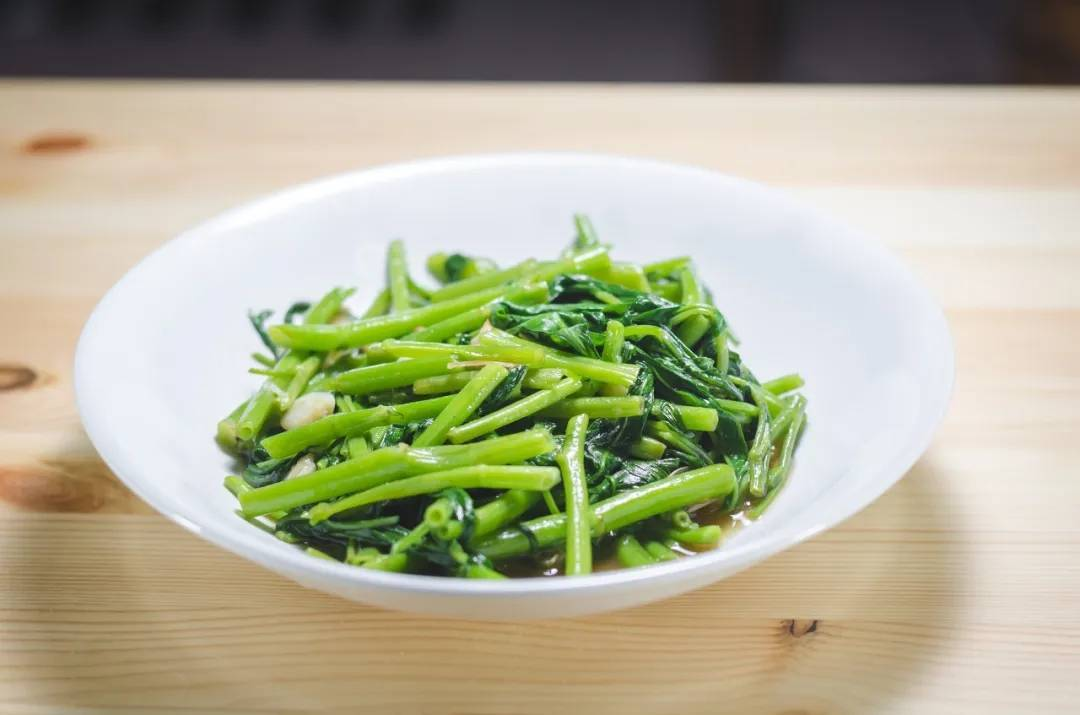• 收起
• 向左旋转
• 向右旋转• 收起
• 向左旋转
• 向右旋转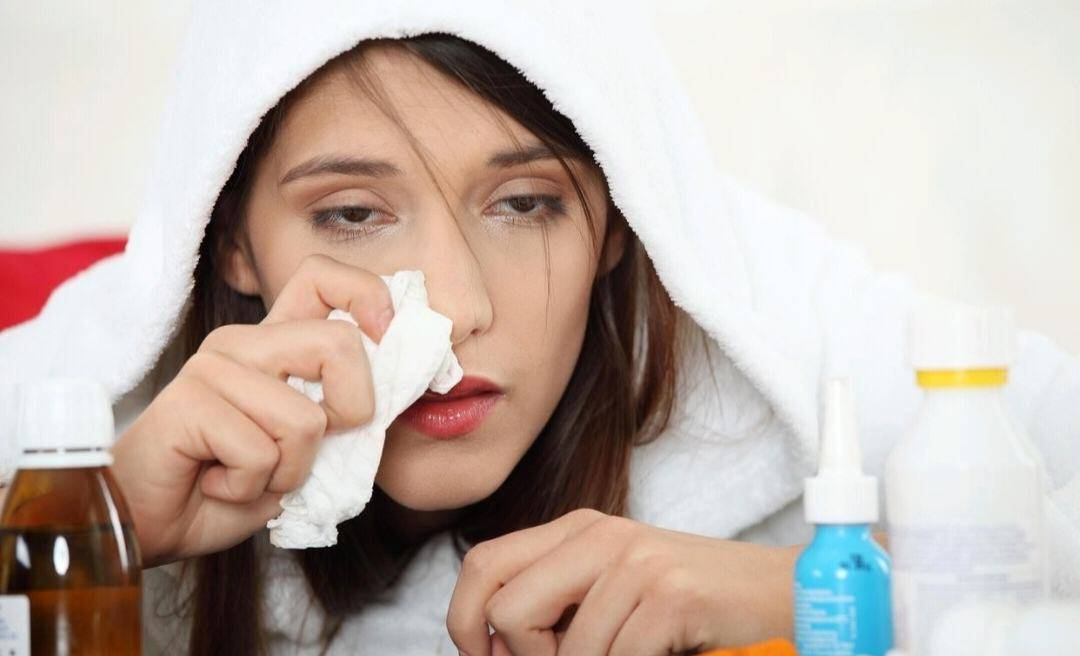• 收起
• 向左旋转
• 向右旋转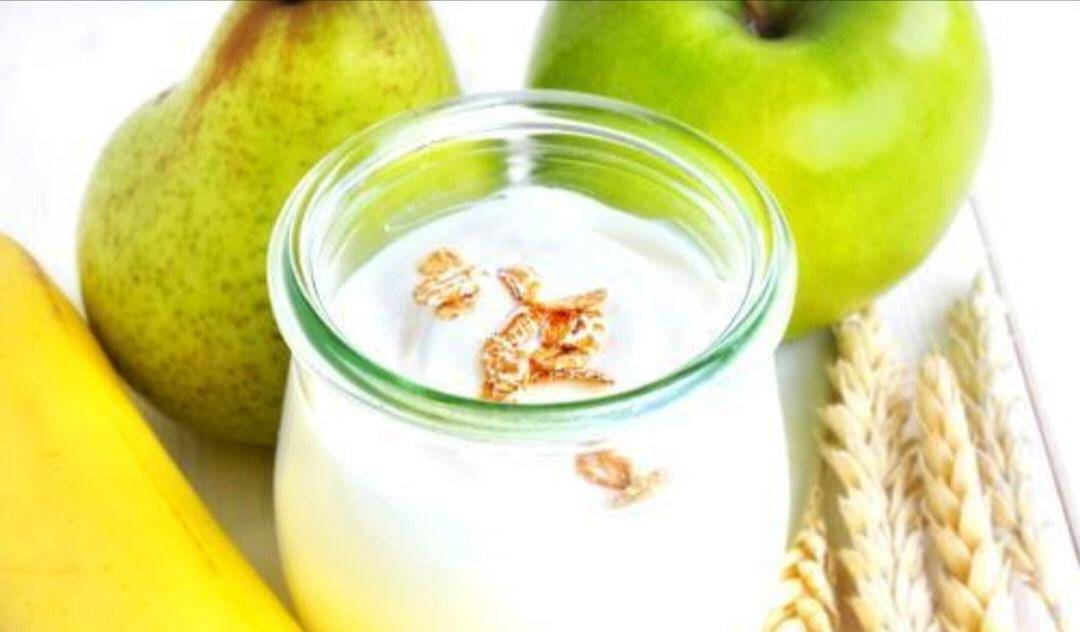• 收起
• 向左旋转
• 向右旋转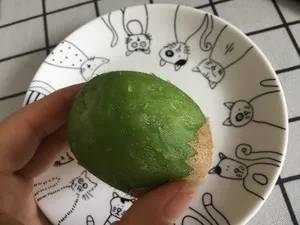• 收起
• 向左旋转
• 向右旋转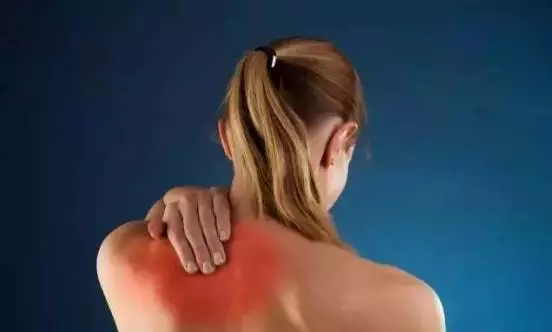• 收起
• 向左旋转
• 向右旋转• 收起
• 向左旋转
• 向右旋转• 收起
• 向左旋转
• 向右旋转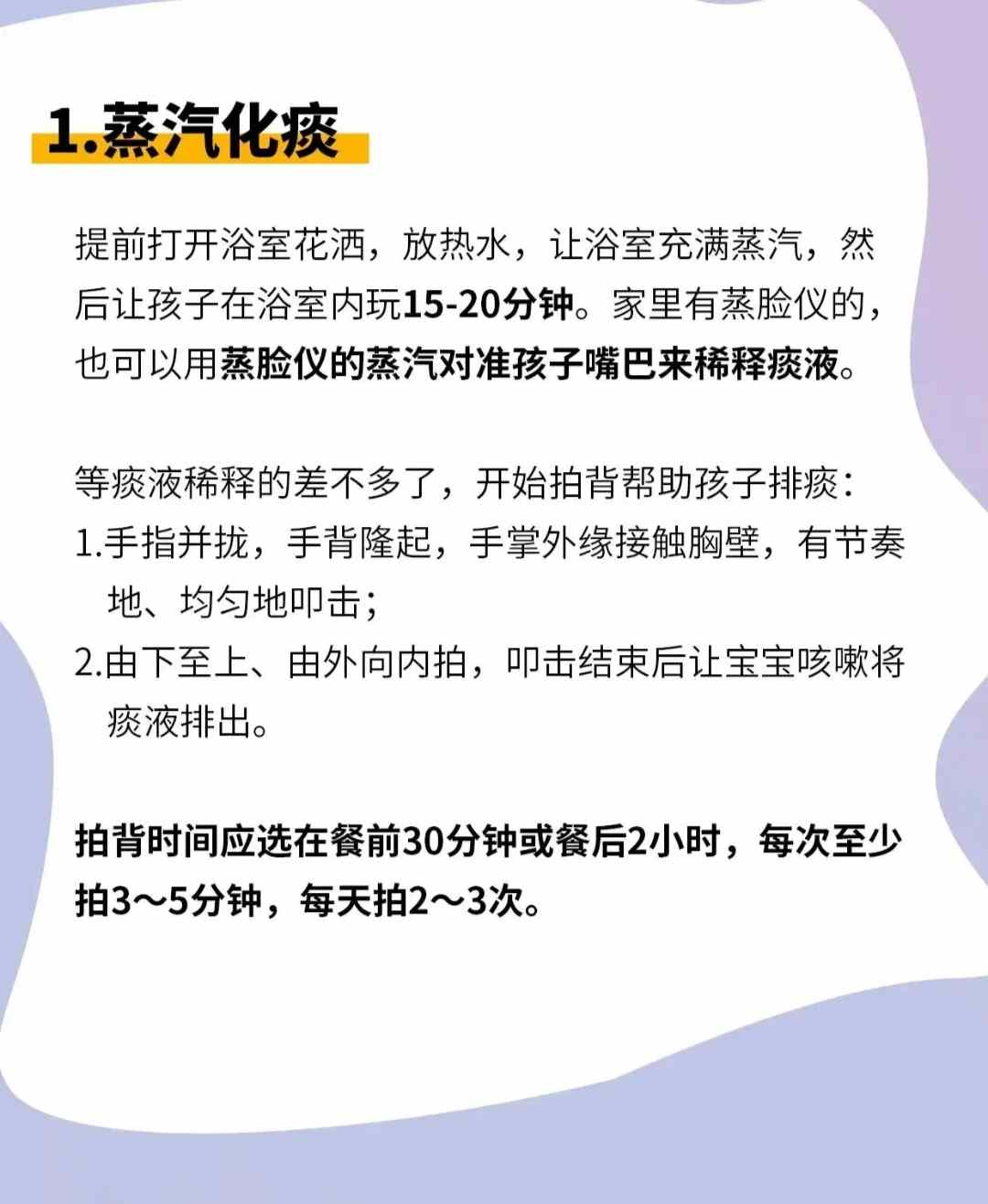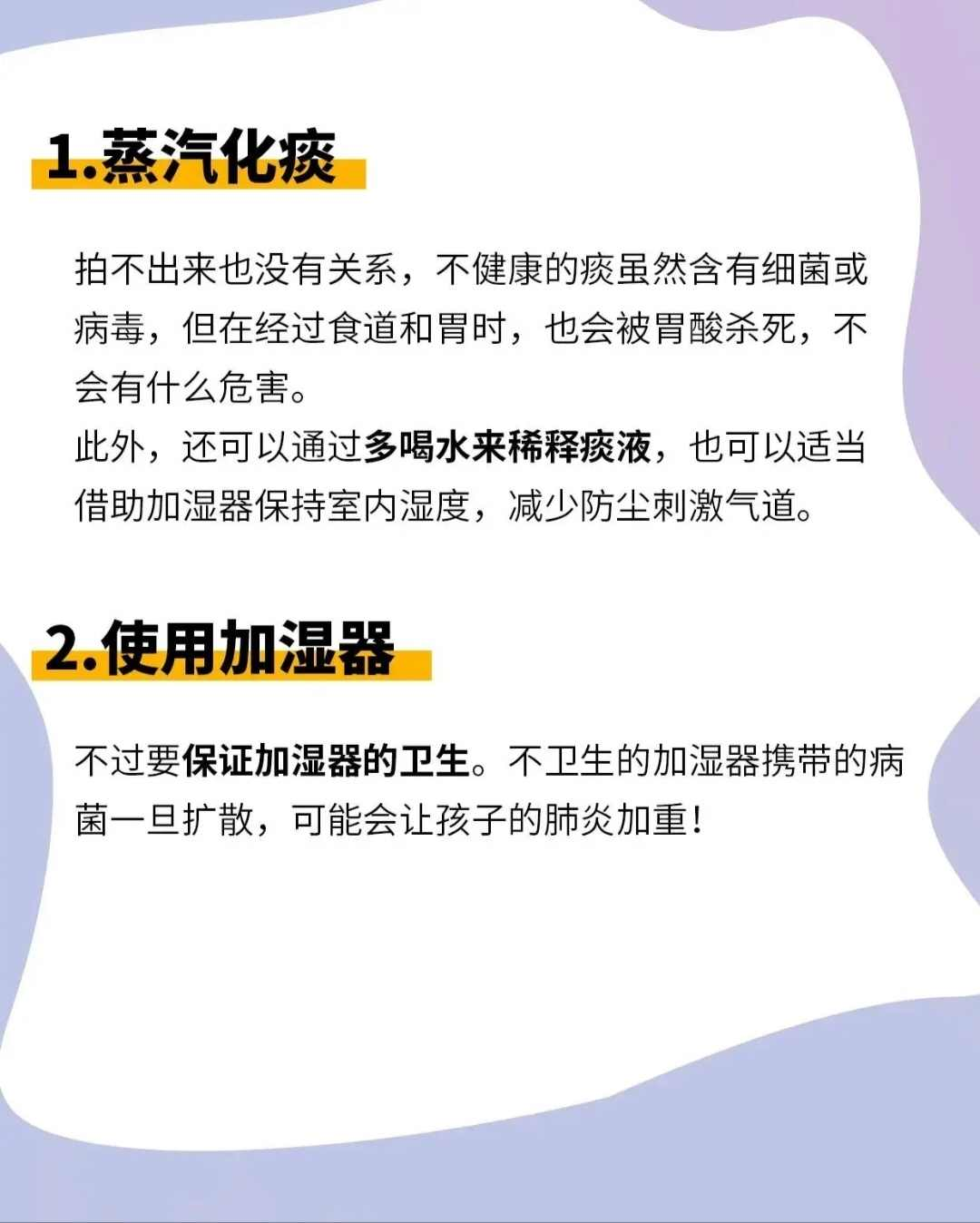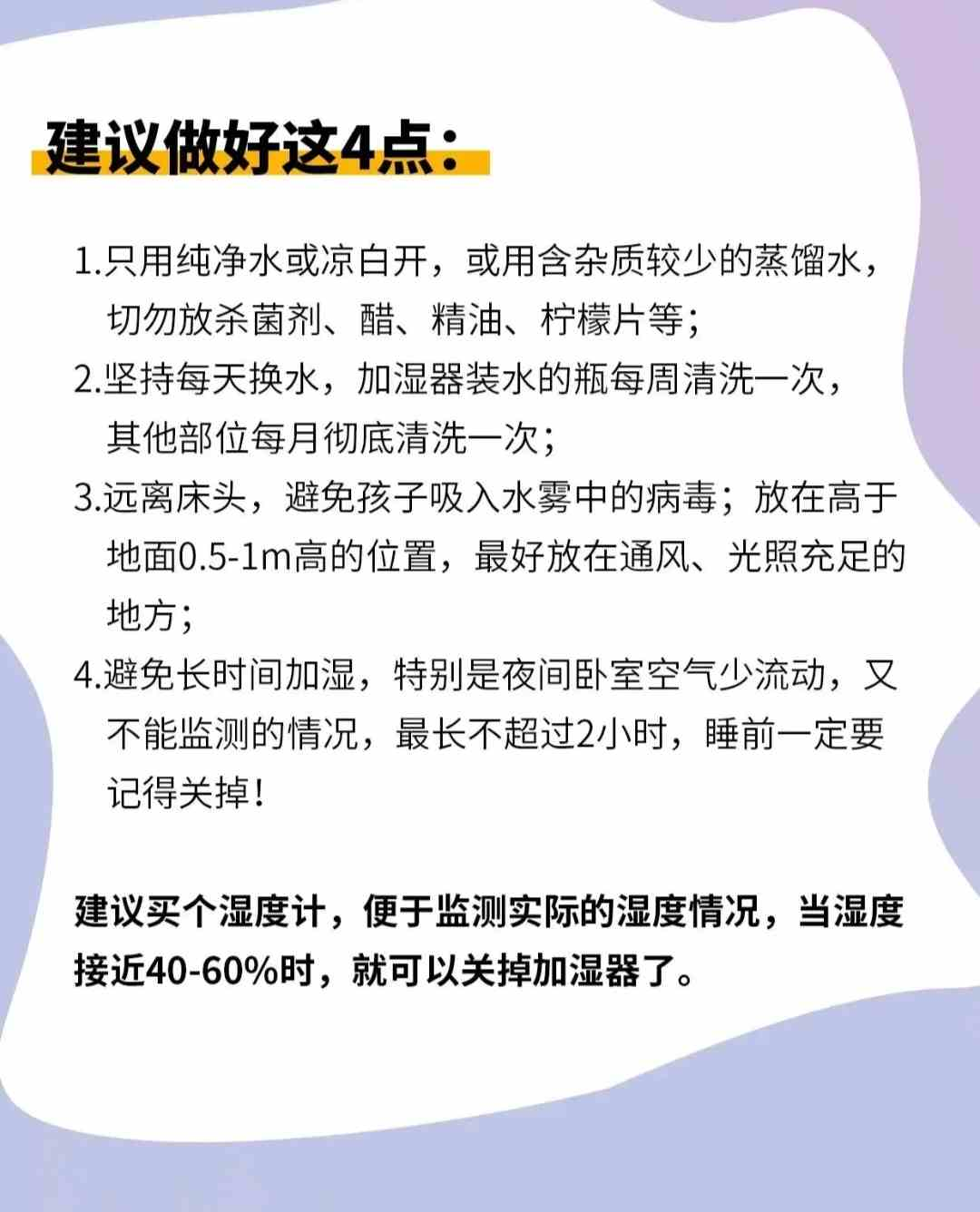• 收起
• 向左旋转
• 向右旋转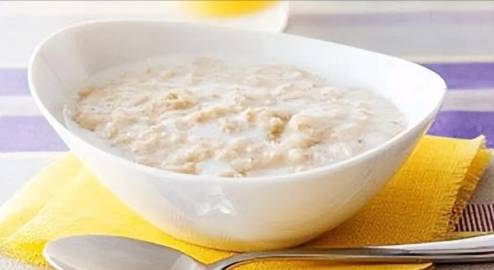• 收起
• 向左旋转
• 向右旋转• 收起
• 向左旋转
• 向右旋转• 收起
• 向左旋转
• 向右旋转• 收起
• 向左旋转
• 向右旋转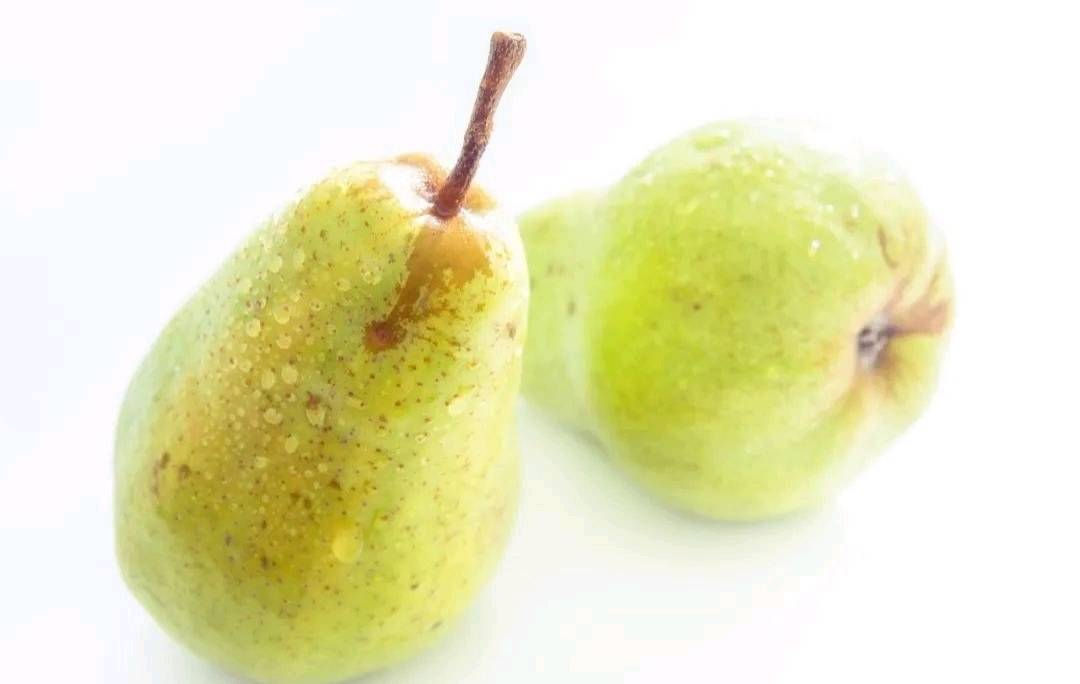• 收起
• 向左旋转
• 向右旋转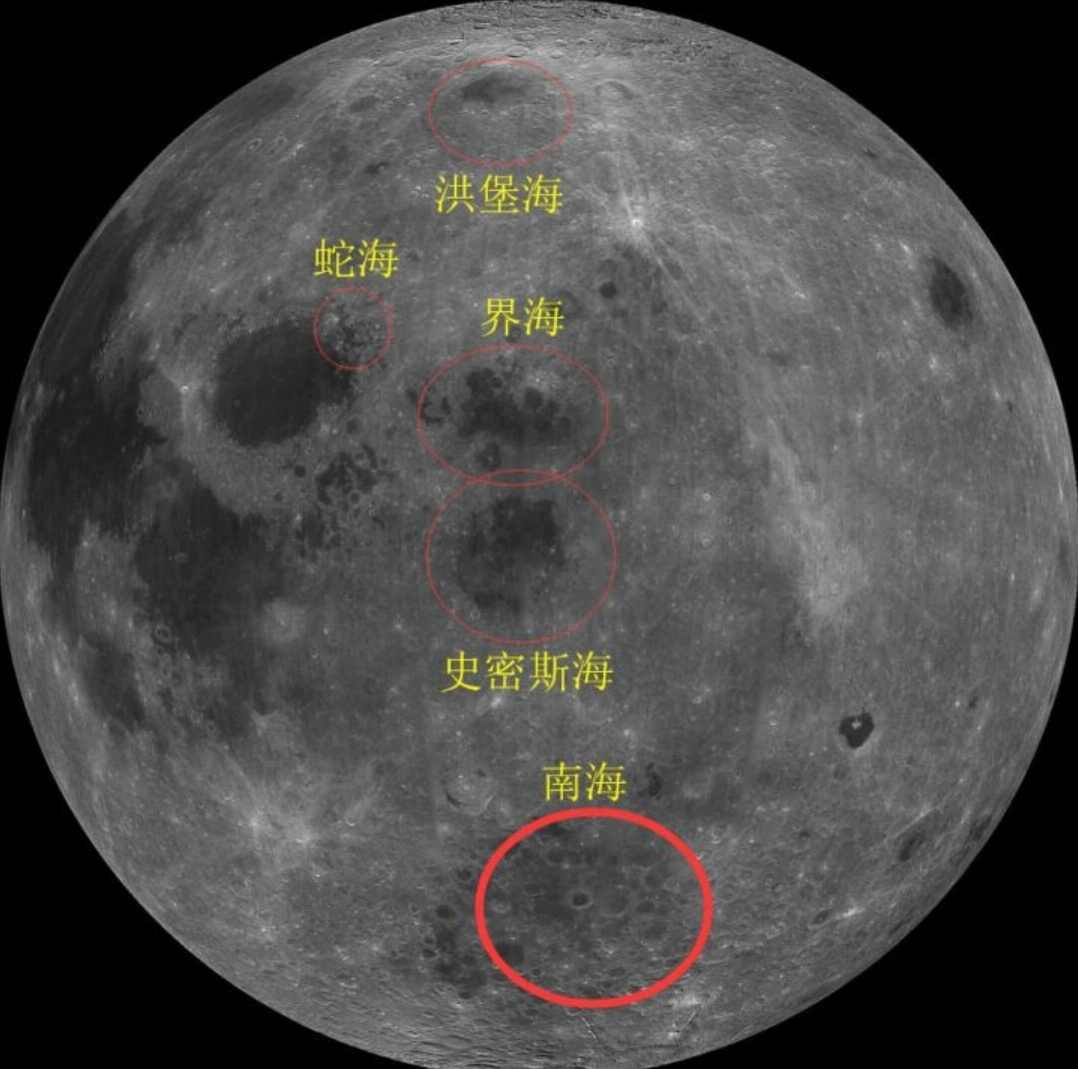• 收起
• 向左旋转
• 向右旋转• 收起
• 向左旋转
• 向右旋转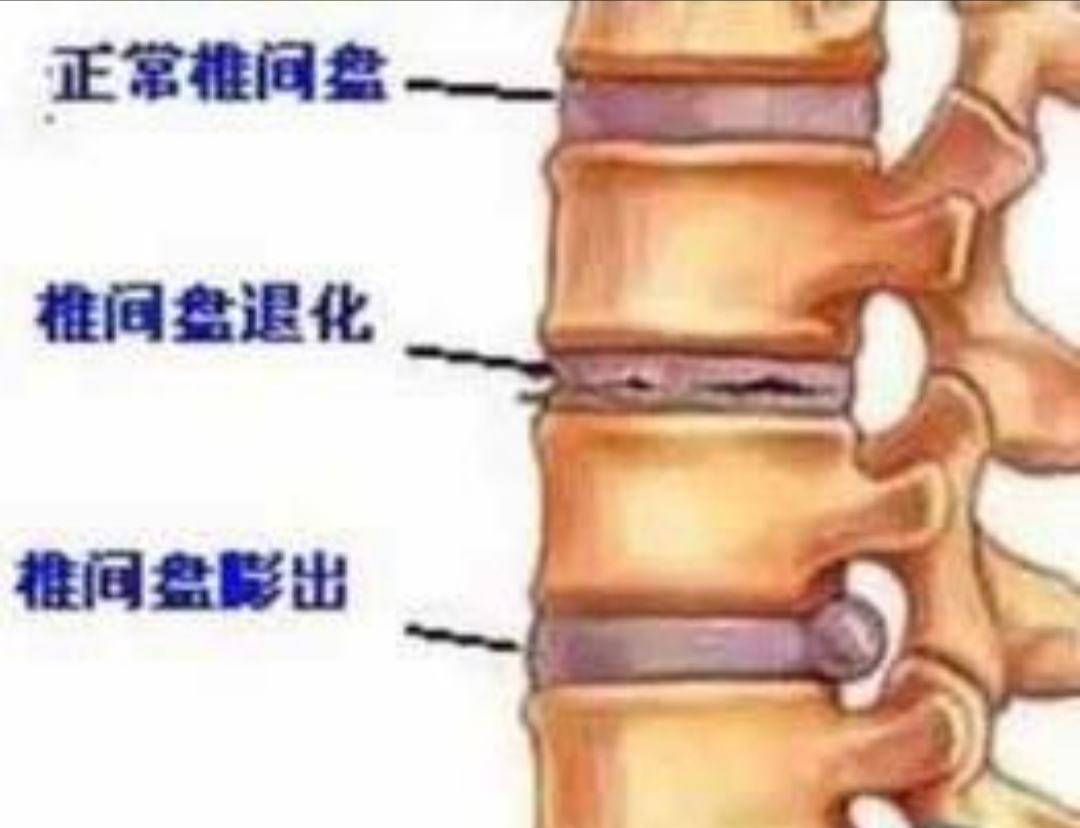• 收起
• 向左旋转
• 向右旋转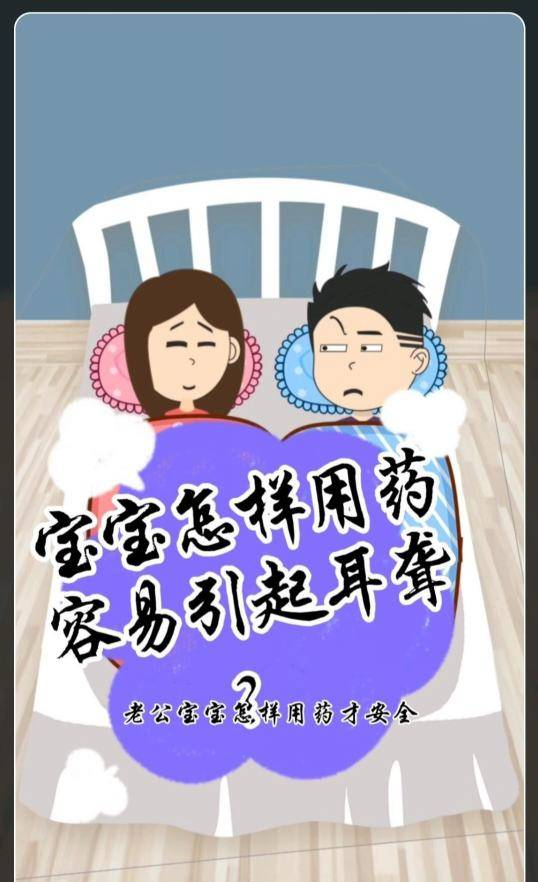• 收起
• 向左旋转
• 向右旋转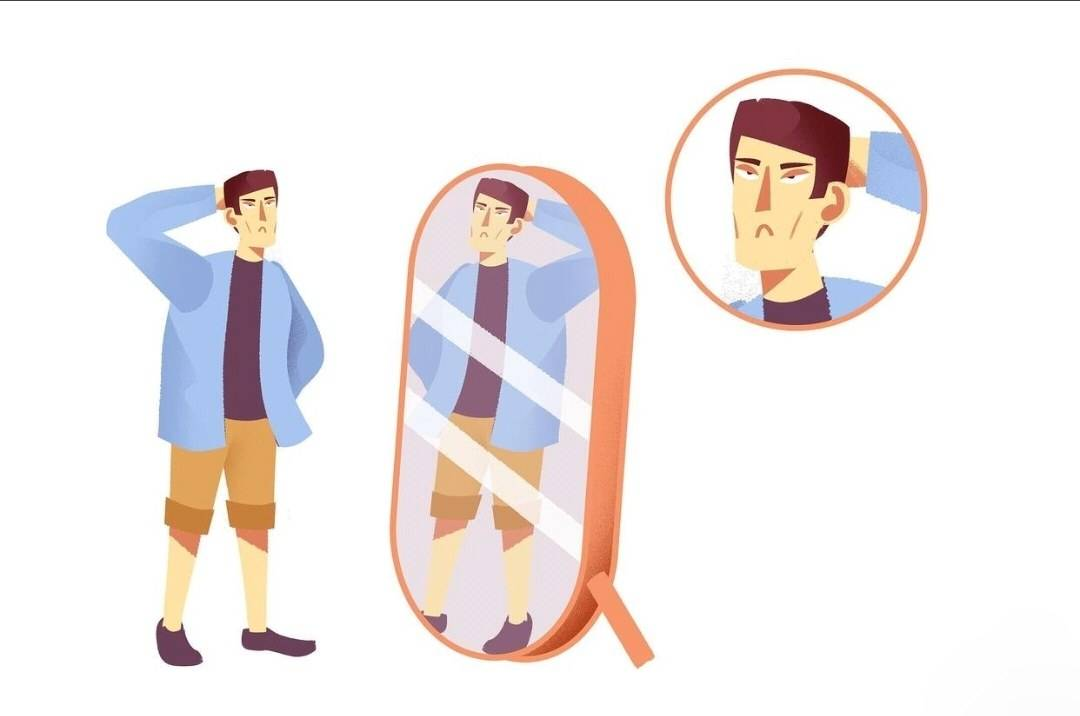• 收起
• 向左旋转
• 向右旋转• 收起
• 向左旋转
• 向右旋转• 收起
• 向左旋转
• 向右旋转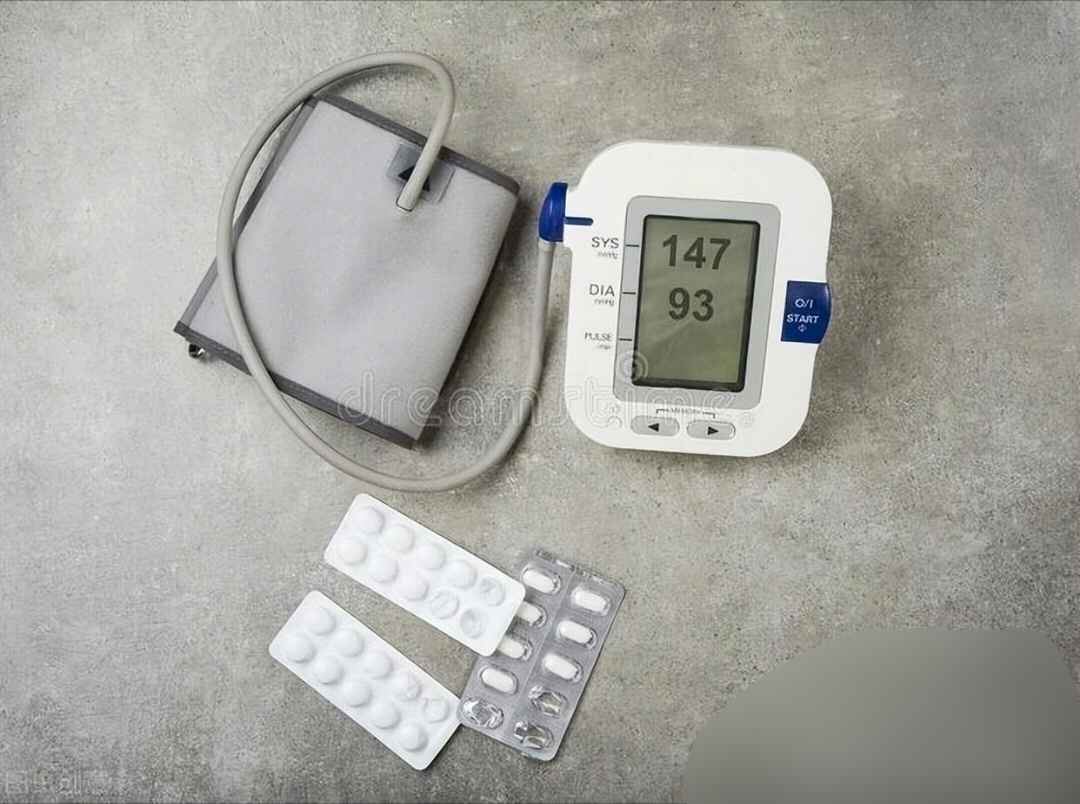• 收起
• 向左旋转
• 向右旋转• 收起
• 向左旋转
• 向右旋转• 收起
• 向左旋转
• 向右旋转• 收起
• 向左旋转
• 向右旋转BB霜和防晒霜先涂哪个？ 先涂防晒霜，后涂BB霜。 要记住，防晒永远是彩妆的第一步。 无论是粉底液、CC霜、素颜霜，还是BB霜，都是在防晒后涂抹的。 BB霜属于底妆类产品，和粉底液类似，主要起到提亮均匀肤色的作用。 只不过质地比粉底液轻薄一些，更加滋润，相对来说，遮瑕效果就没有粉底液那么好。 因此，BB霜非常适合用来化日常淡妆，或者直接当素颜霜使用。 而防晒霜的主要功效是防晒，一般都是乳液质地的，比较滋润。 如果，先涂BB霜，后涂防晒，防晒霜会把底妆溶解，让底妆斑驳卡粉。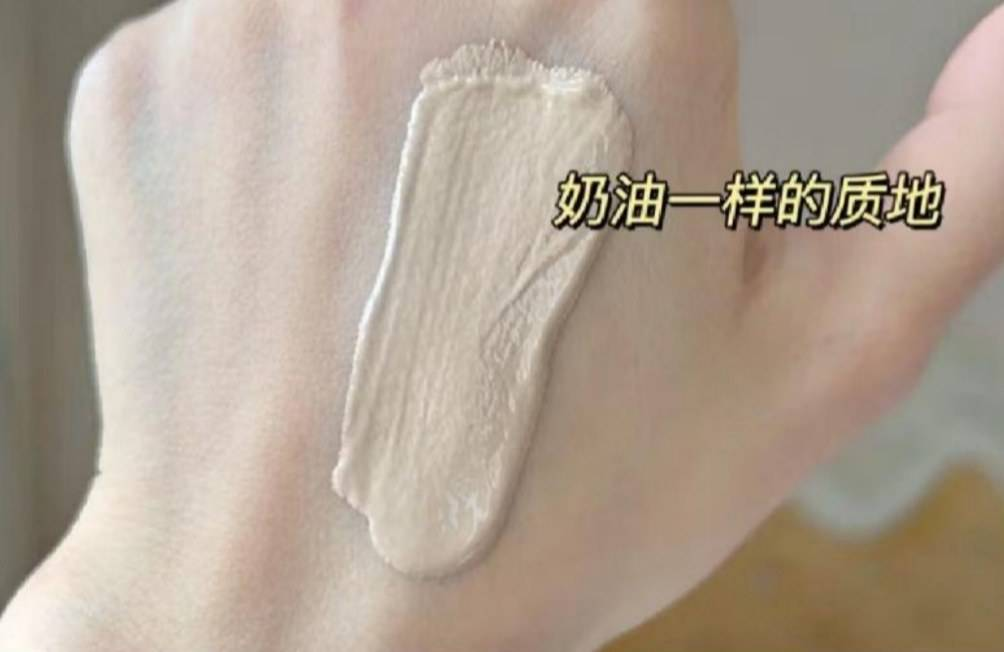• 收起
• 向左旋转
• 向右旋转